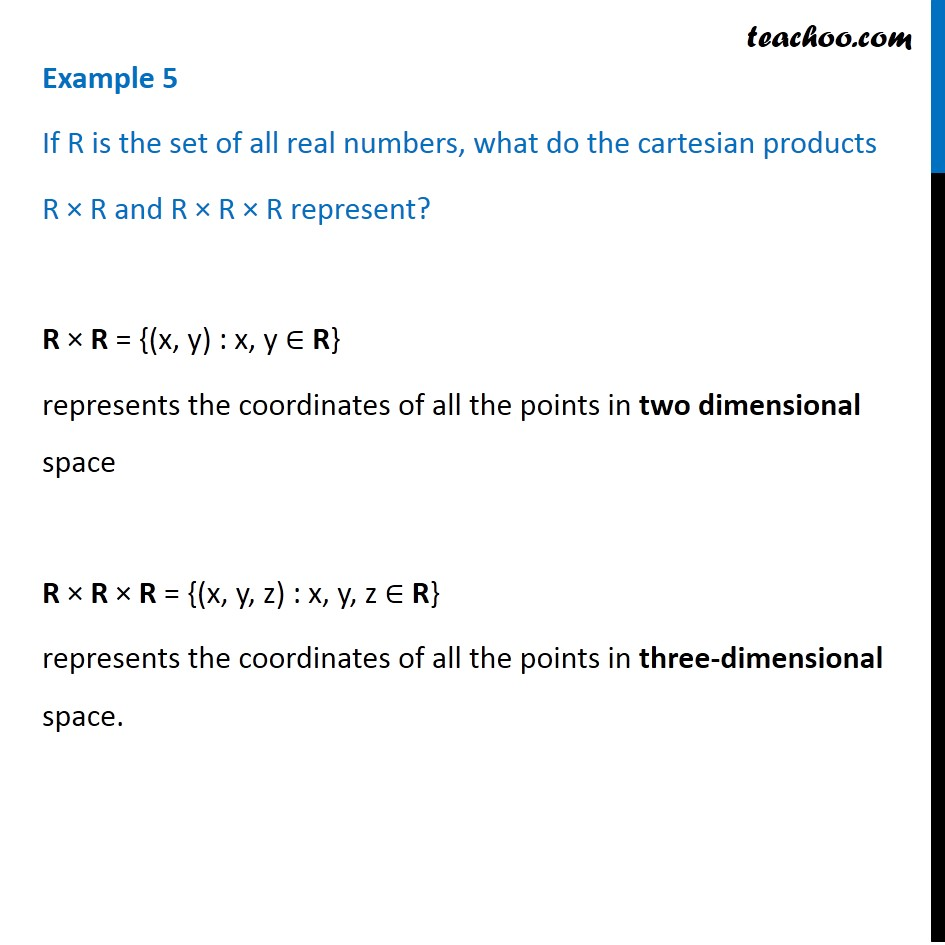Finding Cartesian Product

Chapter 2 Class 11 Relations and Functions
Concept wiseIntroducing your new favourite teacher - Teachoo Black, at only ₹83 per month

### Transcript

Example 5 If R is the set of all real numbers, what do the cartesian products R × R and R × R × R represent? R × R = {(x, y) : x, y ∈ R} represents the coordinates of all the points in two dimensional space R × R × R = {(x, y, z) : x, y, z ∈ R} represents the coordinates of all the points in three-dimensional space.